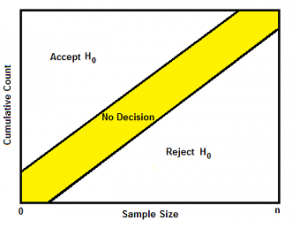# Sequential Probability Ratio Test

## What is a Sequential Probability Ratio Test?

sequential probability ratio test (SPRT) is a hypothesis test for sequential samples.

Sequential sampling works in a very non-traditional way; instead of a fixed sample size, you choose one item (or a few) at a time, and then test your hypothesis. You can either:

If you fail to reach a conclusion, you repeat the sampling and then the hypothesis test. You keep on repeating this process until you have a sound conclusion, so you don’t know the how big your sample will be until you’re finished testing.

Sequential analysis hypothesis testing generally enables a researcher to come to a conclusion with a minimum amount of data. With Wald’s SPRT, the amount of data points required to come to a conclusion can be defined by a random variable, called the sample number Ns. The boundary of the decision region depends on the expected value of this random variable, called the Average Sample Number (ASN). The ASN for the SPRT is lower than all other sequential tests and is usually lower than traditional, fixed-size sampling methods.As in classical hypothesis testing, SPRT starts with a pair of hypotheses, sayfor the null hypothesis and alternative hypothesis respectively. They must be specified as follows:The next step is to calculate the cumulative sum of the log-likelihood ratio, as new data arrive: with, then, for i=1,2,…,The stopping rule is a simple thresholding scheme:

•: continue monitoring (critical inequality)
•: Accept•: Acceptwhere a and b) depend on the desired type I and type II errorsand. They may be chosen as follows:andThe sequential probability ratio test can also be applied in situations where the observations are not independent and identically distributed.